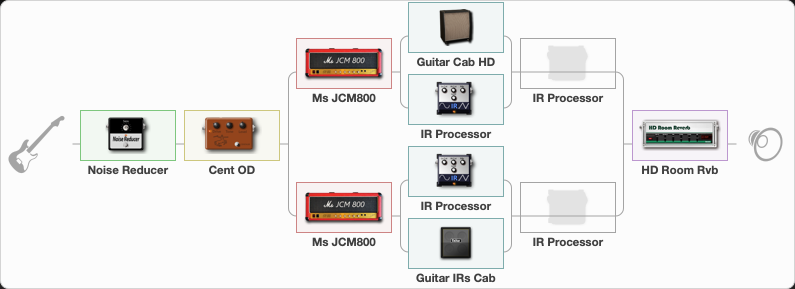Discussion in 'ToneLib-GFX presets' started by grigoriy0192873, Mar 20, 2023.

1. ### grigoriy0192873Active Member

Preset name: Peace sells

Effects chain:Effect: "Noise Reducer" (Dynamics / Filter), active - "yes"
{
"Sens" = 10
"Mode" = Soft
}

Effect: "Cent OD" (Overdrive / Distortion), active - "yes"
{
"Drive" = 40
"Tone" = 80
"Level" = 100
}

Effect: "Splitter" (Dynamics / Filter)
{
"A-Bypass" = Off
"A-Pan" = -25
"A-Level" = 55
"B-Bypass" = Off
"B-Pan" = 25
"B-Level" = 55
"Width" = 0

'A' branch:
{

Effect: "Ms JCM800" (Amp simulators), active - "yes"
{
"Gain" = 40
"Bass" = 40
"Middle" = 70
"Treble" = 50
"Presence" = 50
"Master" = 50
"Level (dB)" = 7
}

Effect: "Splitter" (Dynamics / Filter)
{
"A-Bypass" = Off
"A-Pan" = -25
"A-Level" = 55
"B-Bypass" = Off
"B-Pan" = 25
"B-Level" = 55
"Width" = 0

'A' branch:
{

Effect: "Guitar Cab HD" (Cabinets), active - "yes"
{
"Model" = Marshall 1960A (4x12")
"Mic Model" = Dynamic MD421
"Mic Position" = Middle
"Mic Distance" = Middle
"Low Cut (Hz)" = 80
"Hi Cut (kHz)" = 20.0
"Mix" = 100
"Level (dB)" = 0
}
}
'B' branch:
{

Effect: "IR Processor" (Cabinets), active - "yes"
{
"Low Cut (Hz)" = 0
"Hi Cut (kHz)" = 20.0
"Mix" = 100
"Level (dB)" = 0
}
}
}

Effect: "IR Processor" (Cabinets), active - "no"
{
"IR" = Marshall 1960A 4x12 SM57 +1_00
"Low Cut (Hz)" = 0
"Hi Cut (kHz)" = 14.0
"Mix" = 100
"Level (dB)" = 0
}
}
'B' branch:
{

Effect: "Ms JCM800" (Amp simulators), active - "yes"
{
"Gain" = 50
"Bass" = 50
"Middle" = 50
"Treble" = 50
"Presence" = 50
"Master" = 50
"Level (dB)" = 0
}

Effect: "Splitter" (Dynamics / Filter)
{
"A-Bypass" = Off
"A-Pan" = -25
"A-Level" = 55
"B-Bypass" = Off
"B-Pan" = 25
"B-Level" = 55
"Width" = 0

'A' branch:
{

Effect: "IR Processor" (Cabinets), active - "yes"
{
"Low Cut (Hz)" = 0
"Hi Cut (kHz)" = 20.0
"Mix" = 100
"Level (dB)" = 0
}
}
'B' branch:
{

Effect: "Guitar IRs Cab" (Cabinets), active - "yes"
{
"Model" = Marshall 1960b (4x12")
"Mic Position" = Center
"Mic Distance" = Near
"Low Cut (Hz)" = 60
"Hi Cut (kHz)" = 20.0
"Mix" = 100
"Level (dB)" = 0
}
}
}

Effect: "IR Processor" (Cabinets), active - "no"
{
"IR" = Marshall 1960A 4x12 SM57 +1_00
"Low Cut (Hz)" = 0
"Hi Cut (kHz)" = 14.0
"Mix" = 100
"Level (dB)" = 0
}
}
}

Effect: "HD Room Rvb" (Reverberation), active - "yes"
{
"Time" = 6.6
"PreLPF" = 77
"PreDelay" = 60
"HiDamp" = 65
"LoGain" = -3.5
"Mix" = 25
}

Note: You will need to download and install the ToneLib-GFX software to use the preset.